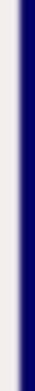homework help forum today's math problem links utilities previous math problem feedback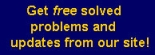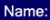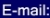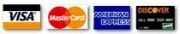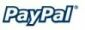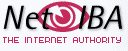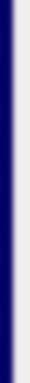# MATH HOMEWORK HELP

Find the domain of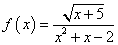Solution: The domain is found by avoiding a division by 0 and roots of negative numbers. In order for the function to be well defined we need:

•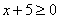•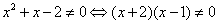From the previous conditions we find that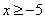and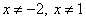Therefore, the domain is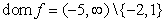MGT - Your source of Math and Statistics Homework Help